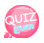# validation float

Let's see how to validate a float.
Use the filter_Var() built-in function.
And use the constant FILTER_VALIDATE_FLOAT as an argument.

## How to validate float using filter_var() function

```filter_Var(float,FILTER_VALIDATE_FLOAT);
```

The first argument uses float and the second uses the constant FILTER_VALIDATE_FLOAT.
As shown in the example, you can change the purpose according to the value of a constant.
Returns true if the float is valid and false.
Here is an example of validating a float:

```<?php
\$int = 1000;

\$checkFloat = filter_Var(\$int, FILTER_VALIDATE_FLOAT);

if(\$checkFloat == true) {
echo "it is float";
} else {
echo "it is not float";
}
?>```

ResultThis time let's test it by typing the wrong float.

```<?php
\$int = "aaa";

\$checkFloat = filter_Var(\$int, FILTER_VALIDATE_FLOAT);

if(\$checkFloat == true) {
echo "it is float";
} else {
echo "it is not float";
}
?>```

이 코드의 결과